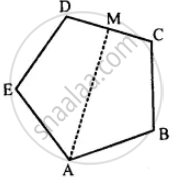# Abcde is a Regular Pentagon. the Bisector of Angle a of the Pentagon Meets the Side Cd in Point M. Show that ∠Amc = 90°. - Mathematics

Sum

ABCDE is a regular pentagon. The bisector of angle A of the pentagon meets the side CD in point M. Show that ∠AMC = 90°.

#### SolutionGiven: ABCDE is a regular pentagon.

The bisector ∠A of the pentagon meets the side CD at point M.

To prove : ∠AMC = 90°

Proof: We know that the measure of each interior angle of a regular pentagon is 108°.

∠BAM =  x 108° = 54°

Since, we know that the sum of a quadrilateral is 360°

∠BAM + ∠ABC + ∠BCM + ∠AMC = 360°

54° + 108° + 108° + ∠AMC = 360°

∠AMC = 360° – 270°

∠AMC = 90°

Is there an error in this question or solution?

#### APPEARS IN

Selina Concise Mathematics Class 8 ICSE
Chapter 16 Understanding Shapes
Exercise 16 (C) | Q 14 | Page 188